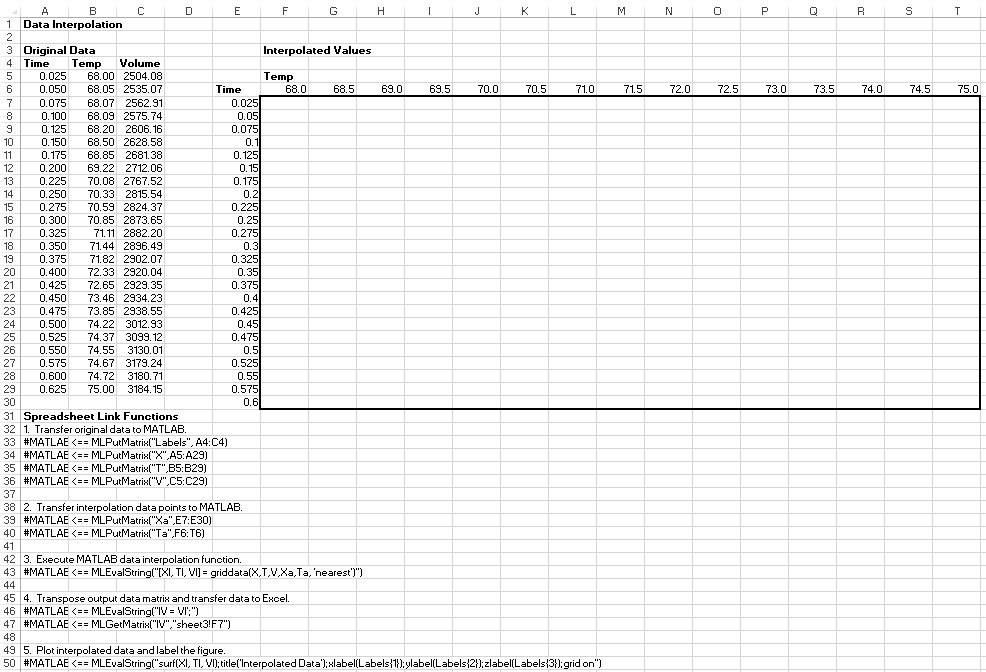Documentation

## Interpolate Thermodynamic Data

This example shows how to interpolate data using Spreadsheet Link™ to invoke MATLAB® functions in Microsoft® Excel®.

The example uses the two-dimensional data-gridding interpolation function `griddata` on thermodynamic data, where volume has been measured for time and temperature values. The `griddata` function finds the volume values underlying the two-dimensional time-temperature function for a new set of time and temperature coordinates.

To organize and display the original data and the interpolated output data, you can use Microsoft Excel worksheets.

Open the `ExliSamp.xls` file and select the Sheet3 worksheet. For help finding the `ExliSamp.xls` file, see Installation.

This worksheet contains measured thermodynamic data in cell ranges `A5` through `A29`, `B5` through `B29`, and `C5` through `C29`. The time and temperature values for interpolation are in cell ranges `E7` through `E30` and `F6` through `T6`, respectively.1. Execute the Spreadsheet Link function that passes the `Time`, `Temp`, and `Volume` labels to the MATLAB workspace by double-clicking the cell `A33` and pressing Enter.

2. Copy the original time data to the MATLAB workspace by executing the function in the cell `A34`. To copy the original temperature data, execute the function in the cell `A35`. To copy the original volume data, execute the function in cell `A36`.

3. Copy the interpolation time values to the MATLAB workspace by executing the function in cell `A39`. To copy the interpolation temperature values, execute the function in cell `A40`.

4. Execute the function in cell `A43`. The `griddata` function performs two-dimensional interpolation that generates the interpolated volume data using the inverse distance method.

5. Transpose the interpolated volume data and copy it to the Excel worksheet by executing the functions in cells `A46` and `A47`. The data fills the cell range `F7:T30`.6. Execute the function in cell `A50`. The MATLAB software plots and labels the interpolated data on a three-dimensional color surface, with the color proportional to the interpolated volume data.To generate different volume values, close the figure and change the measured thermodynamic data in cells `A5:C29`. Then, execute all the Spreadsheet Link functions again. The worksheet updates with new volume rates and MATLAB generates a new figure of the interpolated volume data.